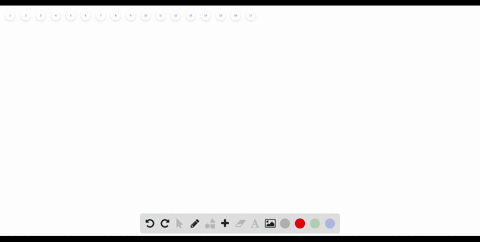Enroll in one of our FREE online STEM bootcamps. Join today and start acing your classes!View Bootcamps### WRITING A FORMULAVrite a formula to ind the numbe…

02:08Case Western Reserve University
Problem 34

# MODELING WITH MATHEMATIGSe loor of the gazebo shown is shaped like a regular decagon.Find the measure of each interior angle of the regular decagon. Then I nd the measure of each exterior angle.

## Discussion

You must be signed in to discuss.

## Video Transcript

So this question There's a gazebo whose throw the shaped like a regular check up on. We need to find the measure of each interior angle. I just did not like this and its exterior. No, since a deck of onions 10 site. We're going to use the included angle formula, which is 1 80 times and minus two, which in this case is it to find the total sum. And this is going to be equal to 1440 It is. Since this is the sum of interior angles, we just divide this by 10 because it has an angle. 10 Indeedy Rangel's to get 1 44 Dixie similar differently. Exterior angles you need to use the extended angle pushed it. It says that the measure off individual exterior angles is nothing but a 360 divided by the number off sites. And in this case, this is 10 and therefore the exterior. The landing off each exterior angle. It's only 60 years on the beach, indeedy. It angle is I'm within 40 40